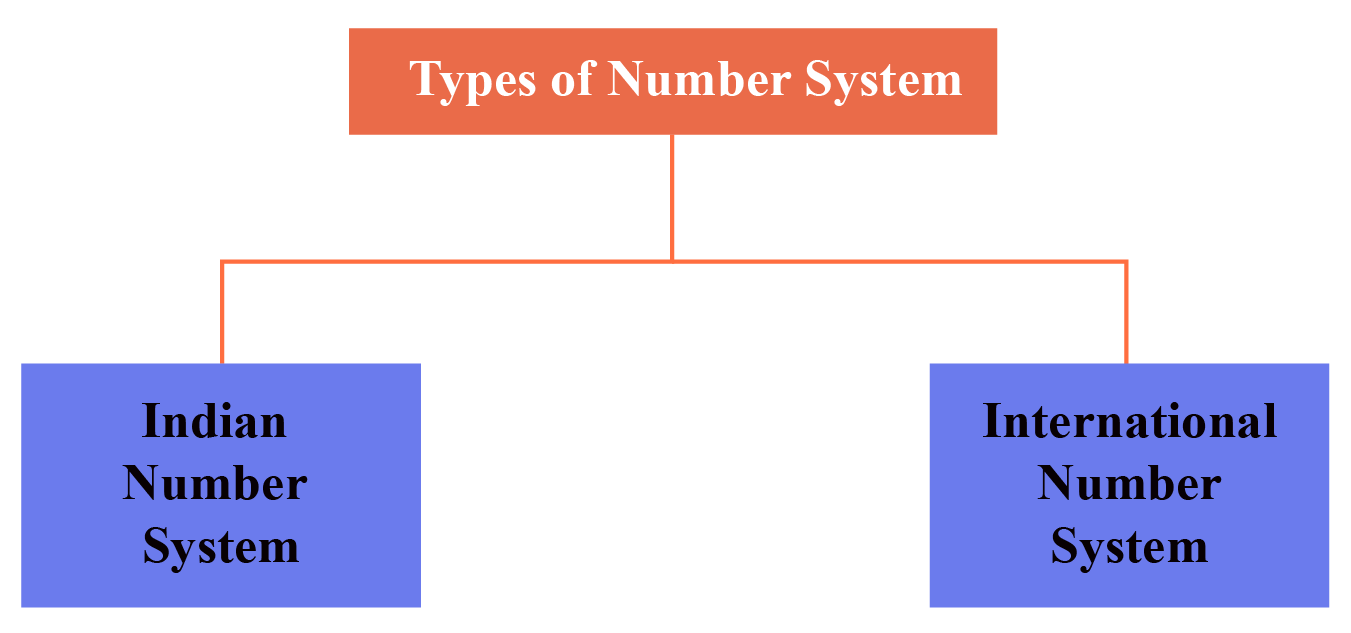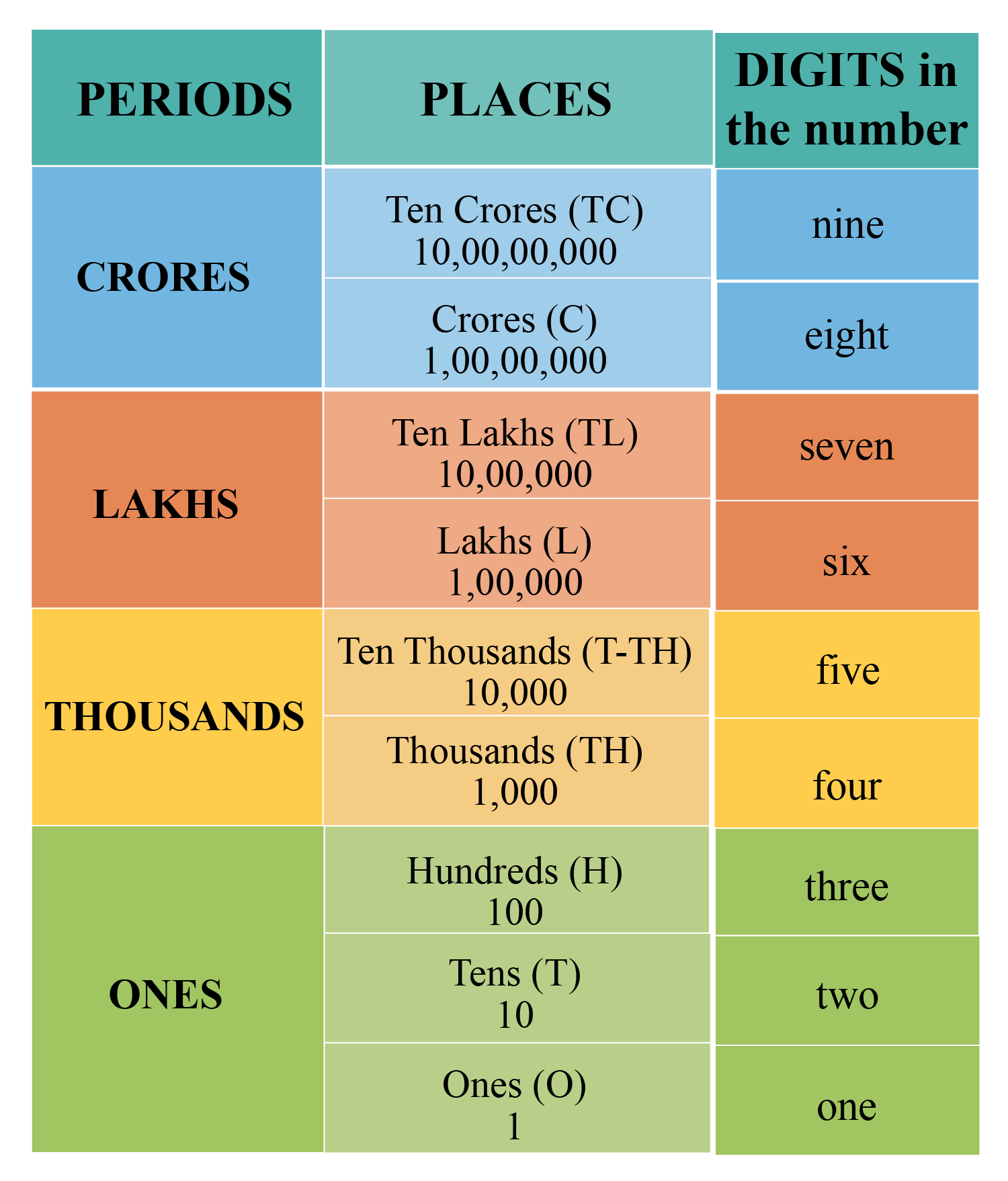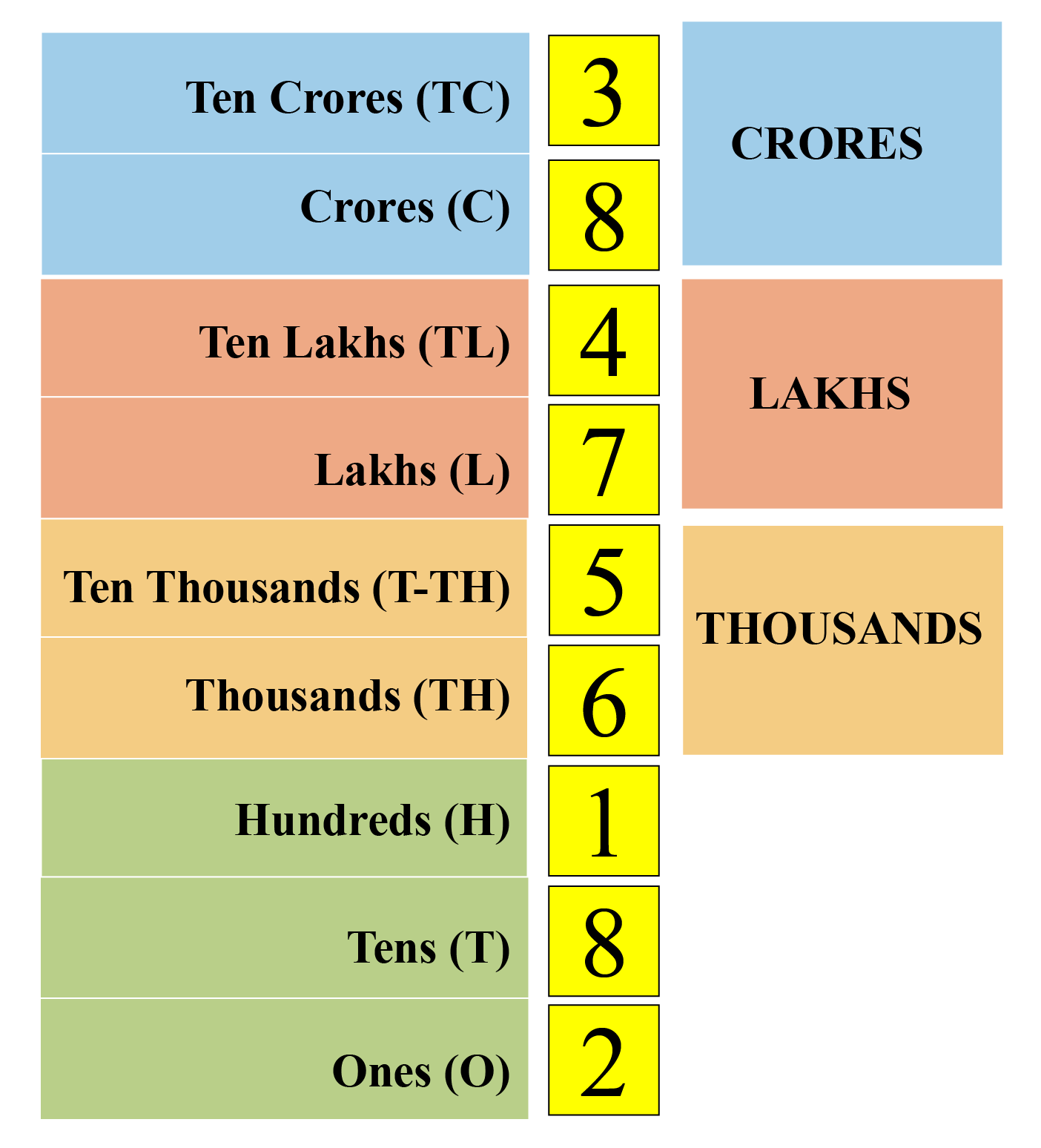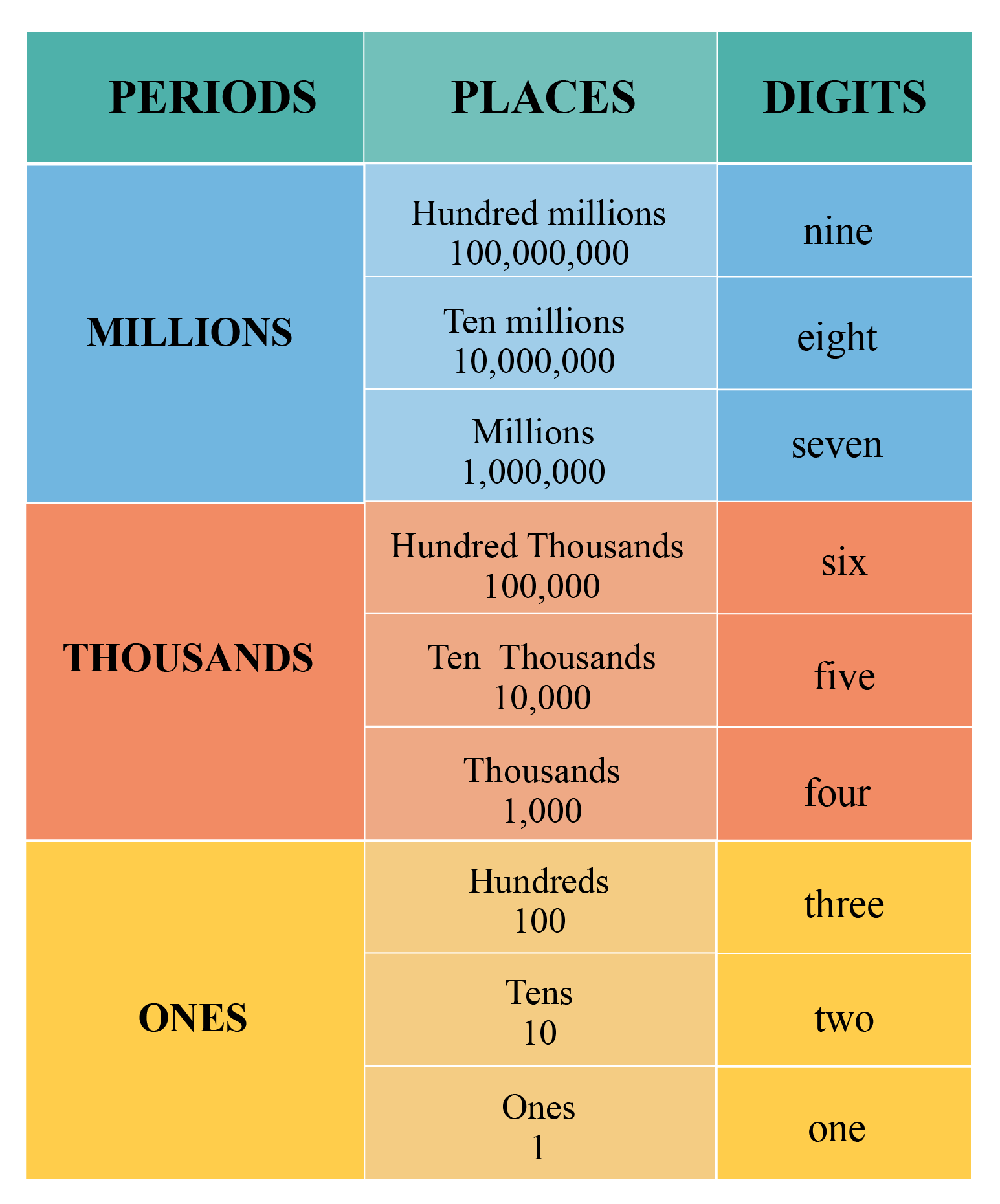# Indian Numeral System

Go back to  'Number Systems'

In this mini-lesson, we will explore the world of the Indian Numeral System. We will learn about the difference between the Indian and International Numeral Systems and the place value of digits in numbers according to the Indian system. So what are we waiting for? Let’s begin!

Long before numbers were invented, a unique mark was made on each item being counted. This was a complex system for writing large numbers.

People realized that there was a need to adopt a standard system of counting. This is how the numeral system came into existence.

Different numeral systems were used across different countries and periods.

We know that numbers in mathematics are used for counting, measurements, and comparisons.

A number system contains numerals which are used to represent the numbers.

There are two types of number systems: Indian numeral system and International numeral system.## Lesson Plan

 1 What is Indian Numeral System? 2 Solved Examples on Indian Numeral System 3 Interactive Questions on Indian Numeral System 4 FREE Downloadable Resources on Indian Nmberal Systems

## Free PDFs for Offline Revision

Get your copy of Indian Numeral System E-book along with Worksheets and Tips and Tricks PDFs for Free!

## What is the Indian Numeral System?

When we count with ones, tens, hundreds, thousands, ten thousand, lakhs, ten lakhs, and crores, we use the Indian system of numeration.

Look at the following table that shows the periods, places and digits in a number.The ONES period consists of 3 places: Ones, tens and hundreds.

The THOUSANDS period comprises 2 places: Thousands and ten thousands.

The LAKHS period comprises 2 places: Lakhs and ten lakhs.

The CRORES period comprises 2 places: Crores and ten crores.

Generate a number in the given simulation and drag the pointers to determine the place value of each digit.

Let's play along and learn!

## Unlock the Mystical World of Indian Numeral System!

You might be interested in reading these mini lessons for a better understanding of the Indian Numeral System.

Explore them and deep dive into the mystical world of the Indian numeral system.

Click on a mini-lesson to explore further!

Numbers Up To 6-digits
Numbers Up To 7-digits
Numbers Up To 4-digits
Indian Place Value Chart
Numbers Up To 3-digits

## What Are Indian Numerals from 1 to 10?

Jagat owns a huge farm.

He sold his entire wheat harvest for Rs. 384756182.Let us try to read this big number, "384756182", using the Indian numeral system.

First, we will place it in the Indian place value chart.

Starting from the right-most number, this number represents the ones digit.It is easy to read any large number if we place the number in the place value chart of the Indian numeral system.

Therefore, the number is "Thirty eight crore forty seven lakh fifty six thousand one hundred and eighty two".

Let us see how the 6 and 7 digits numbers are written in the Indian Numeral System.Think Tank
1. How many thousands are there in one lakh?
2. What is one more than the greatest 7-digit number?
3. Write the smallest 3 digit number which does not change if the digits are reversed.

## What Is the Difference between Indian Numeral System and International Numeral System?

The International numeral system is used worldwide.

This is Aaron.

He says that he has bought a house for 4 million rupees.But, how much is one million?

Let us see.

When we count in terms of ones, hundreds, thousands, ten thousands, hundred thousands, millions and billions, then we are referring to the International numeral system.

The following table shows the periods, places, and digits in a number for the International numeral system.Thus, in one million, there are 6 zeros and 7 digits.

Now, let's learn to read and write a number using the International numeral system.

We will write the numbers in the standard form using commas and read the numbers using periods.

For example:

Rewrite the number $$1347823$$ in the International numeral system using commas.

How will you read this number?

Solution:

In the International numeral system, we begin from the right and insert commas after every three digits.

We divide the number in periods and read the period separately.

Standard Form: $$1,347,823$$

Number Name: One million three hundred forty seven thousand eight hundred and twenty three.

Thus, the main difference in Indian and international numeration system is the placement of the commas.Tips and Tricks

After comparing the Indian and International Numeral System, we have:

• 100 thousands = 1 lakh
• 1 million = 10 lakhs
• 10 millions = 1 crore
• 100 millions = 10 crores

## Solved Examples

Let us solve a few examples to understand the Indian numeral system.

 Example 1

Rewrite the number 2687393 by placing the comma as per the Indian numeral system.

Solution:

In the Indian numeral system, we use commas to mark thousands, lakhs and crores.

We insert a comma after each period.

Starting from the right side of the number, the first comma is inserted after the hundreds digit.

The second comma is inserted after the thousands period, that is after 2 places from the first comma.

The third comma is inserted after the lakhs period.

This is how we insert the commas in the Indian numeral system.

 $$26,87,393$$
 Example 2

How will the number 2,34,32,829 be read out according to the Indian numeral system?

Solution:

The number 2,34,32,829 will be read as "Two crore thirty four lakh thirty two thousand eight hundred and twenty nine".Challenging Questions
1. What is the place value of 5 in the number 3456781 according to the Indian and International numeral system?
2. How many millions are there in one billion?

## Interactive Questions

Here are a few activities for you to practice. Select/Type your answer and click the "Check Answer" button to see the result.

## Let's Summarize

The mini-lesson was aimed at helping you learn about the Indian numeral system. Hope you enjoyed learning about them and exploring the difference between the Indian and International Numeral systems.

We would love to hear from you. Drop us your comments in the chat and we would be happy to help.

At Cuemath, our team of math experts is dedicated to making learning fun for our favorite readers, the students!

Through an interactive and engaging learning-teaching-learning approach, the teachers explore all angles of a topic.

Be it worksheets, online classes, doubt sessions, or any other form of relation, it’s the logical thinking and smart learning approach that we at Cuemath believe in.

## 1. What are the Indian numerals?

Indian numerals is a set of 10 symbols: 0, 1, 2, 3, 4, 5, 6, 7, 8 and 9. These symbols came into existence during 6th or 7th century in India.

## 2. How do you write numbers in the Indian system?

First put the number in the Indian Place Value Chart. While writing a number in Indian numeral system, separate the period using commas.

Indian Numeral System -Ebook
Ebook
Indian Numeral System -Tips
Indian Numeral System

More Important Topics
Numbers
Algebra
Geometry
Measurement
Money
Data
Trigonometry
Calculus